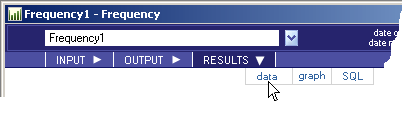# Frequency - RESULTS - Data - Teradata Warehouse Miner

## Teradata Warehouse Miner User Guide - Volume 1Introduction and Profiling

Product
Release Number
5.4.4
Published
July 2017
Language
English (United States)
Last Update
2018-05-03
dita:mapPath
wbc1492033894304.ditamap
dita:ditavalPath
ft:empty
dita:id
B035-2300
Product Category
Software
1. On the Frequency dialog box, click on RESULTS.
2. Click on data (note that the RESULTS tab will be grayed-out/disabled until after the analysis is completed).
Frequency > Results > DataResults data, if any, is displayed in a data grid as described in RESULTS Tab.

The following is a description of the results returned by the analysis, depending on the options selected. If an output table is created, the columns in bold below comprise the Unique Primary Index (UPI) of the output table.

Table Created Without Cross-Tabulation or Pair-wise Frequencies Options
Name Type Definition
xtbl VARCHAR (30) Table that the frequency variable resides in, as specified by the “table” parameter.
xcol VARCHAR (30) Column name representing the frequency variable, as specified by the “column” parameter.
xval VARCHAR (256) Distinct values of the frequency variable.
xcnt FLOAT Count of the number of rows that the frequency variable is equal to the distinct value specified in xval.
xpct FLOAT Percentage of the total records where the frequency variable is equal to the distinct value specified in xval.
xmin_COL FLOAT If the Statistics Columns option is selected, then this column is created and represents the minimum value of the column specified for the given frequency value (xval).

Not available if the Include cumulative measures and rank option is selected.

xmax_COL FLOAT If the Statistics Columns option is selected, then this column is created and represents the maximum value of the column specified for the given frequency value (xval).

Not available if the Include cumulative measures and rank option is selected.

xmean_COL FLOAT If the Statistics Columns option is selected, then this column is created and represents the mean value of the column specified for the given frequency value (xval).

Not available if the Include cumulative measures and rank option is selected.

xstd_COL FLOAT If the Statistics Columns option is selected, then this column is created and represents the standard deviation of the column specified for the given frequency value (xval).

Not available if the Include cumulative measures and rank option is selected.

xcum_cnt FLOAT A running cumulative count of the number of rows that the frequency variable is equal to the distinct value specified in xval. The Include cumulative measures and rank option must be selected to get this result column.

Not valid if the Columns for Statistical Analysis option is selected.

xcum_pct FLOAT A running cumulative percentage of the total records where the frequency variable is equal to the distinct value specified in xval. The Include cumulative measures and rank option must be selected to get this result column.

Not valid if the Columns for Statistical Analysis option is selected.

xrank FLOAT The rank, with respect to all frequencies, where the frequency variable is equal to the distinct value specified in xval. The Include cumulative measures and rank option must be selected to get this result column.

Not valid if the Columns for Statistical Analysis option is selected.

With Cross-Tabulation Option Selected
Name Type Definition
COL Input Column Type Column name that the crosstab frequency will be calculated, as specified by the “column” parameter. Multiple columns, if specified, will be created or displayed as the column name.
xcnt FLOAT Count of the number of rows that the frequency variable is equal to the distinct value specified in xval.
xpct FLOAT Percentage of the total records where the frequency variable is equal to the distinct value specified in xval.
xcum_cnt FLOAT A running cumulative count of the number of rows that the frequency variable is equal to the distinct value specified in xval.

The Include cumulative measures and rank option must be selected to get this result column.

xcum_pct FLOAT A running cumulative percentage of the total records where the frequency variable is equal to the distinct value specified in xval.

The Include cumulative measures and rank option must be selected to get this result column.

xrank FLOAT The rank, with respect to all frequencies, where the frequency variable is equal to the distinct value specified in xval.

The Include cumulative measures and rank option must be selected to get this result column.

With Pair-wise Frequencies Option Selected
Name Type Definition
xtbl VARCHAR (30) Table that the frequency columns reside in, as specified by the “table” parameter.
col1 VARCHAR (30) Column representing the first frequency variable, as specified by the “column” parameter.
val1 VARCHAR (256) Distinct values of the first frequency column.
col2 VARCHAR (30) Column representing the second frequency variable, as specified by the “column” or, alternatively, “column2” parameter.
val2 VARCHAR (256) Distinct values of the second frequency column.
xcnt FLOAT Count of the number of rows that the pair-wise combination of frequency columns are equal to the distinct value specified in val1 and val2, respectively.
xpct FLOAT Percentage of the total records that the pair-wise combination of frequency variables are equal to the distinct value specified in val1 and val2, respectively.